• 提出了用具有树特征的图结构定义类人机器人数据结构方法，应用深度优先搜索算法对其进行遍历，并用螺旋理论和链乘法则来描述机器人姿态，构建出基于Matlab软件仿真平台。该平台引用零力矩点ZMP理论作为机器人...
• 背景知识：图的存储、遍历及其应用图的最短路径等。 目的要求： 掌握图的存储、构建、搜索等操作和应用，能用最短路径及其搜索等算法编制较综合性的程序，求解最优路线问题，进行程序设计、数据结构和算法设计等...
目录

问题：

代码1（这是题目给的代码）

解析：

功能1：

功能2：

代码

代码2（自己写的）

前言

整体思路及运行情况

代码

问题：

背景知识：图的存储、遍历及其应用，图的最短路径等。

目的要求：

掌握图的存储、构建、搜索等操作和应用，能用最短路径及其搜索等算法编制较综合性的程序，求解最优路线问题，进行程序设计、数据结构和算法设计等方面的综合训练。

实验内容：

1．任务：设计一个城市交通咨询模拟系统，利用该系统实现至少两种最优决策：最短路程到达、最省时到达等线路规划。

2．内容：

用户驾车出行由于出行目的的不同对道路路线选择的要求也有不同。例如，有的希望在途中的路程尽可能短，有的则可能希望路程中时间最短。为了能满足广大旅客的需求，编制一个城市交通咨询模拟系统，选取城市部分位置、道路抽象为程序所需要图的顶点和边，并以城市道路长度（路程），道路的某时段的速度等信息作为图结点中的弧信息，为旅客提供这两种最优决策的交通咨询。

实验说明：

1．输入和输出：

（1）输入形式：

构建图时，输入顶点、弧涉及的信息，包括：起始地、目的地、长度、该弧此时间段的平均速度等信息；
用户或者客户要输入出发地和目的地，并选择何种最优决策的路线规划。
（2）输出形式：根据用户需求输出对应信息

输出最短路程所需要的路线信息和最短路程；
输出最短时间所需要的路线信息和最短时间。
2．实验要求：

实现一个简单的交互式界面，包括系统菜单、清晰的输入提示等。
根据输入的交通图数据，以图形化形式把交通图显示在屏幕上。
以图形化形式把最优路线显示在屏幕上。

代码1（这是题目给的代码）

解析：

这个是实验指导书上的源码，挺好用

功能1：

首先建立三个文本文件count文件里面存的地方的个数，map文件里面存的是一个点到另一个点的距离，map_speed文件里面存的是 一个点到另一个点的时间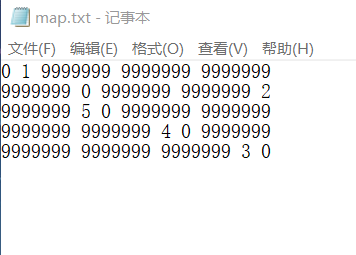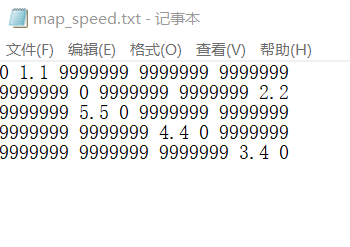看map里面的1，处于第1行第2列，就是第一个点到第二个点的距离是1，

map_speed的1.1就是第1个点到第2个点的花费的时间是1.1

这是我用的测试点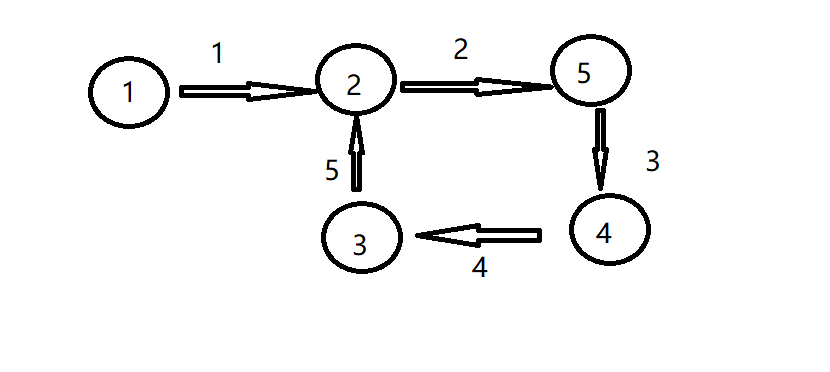下面正式开始测试，首先你要改一下代码中的文件的路径，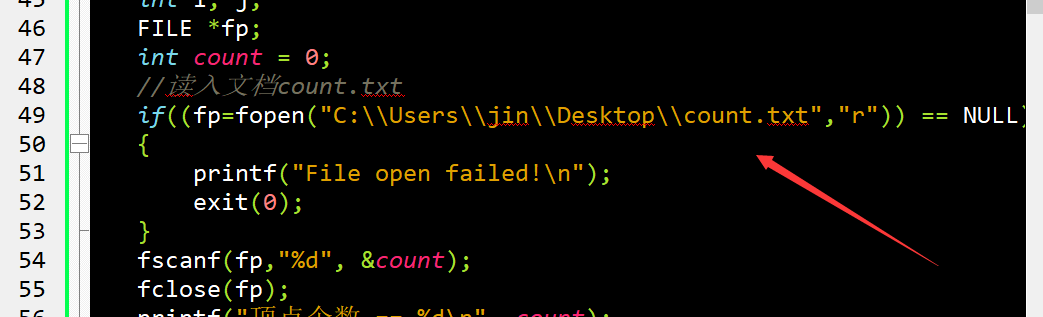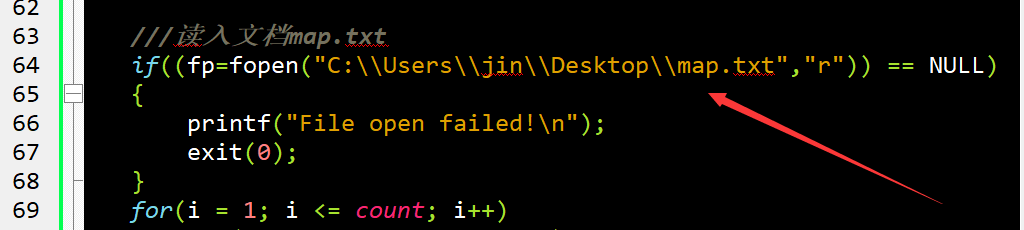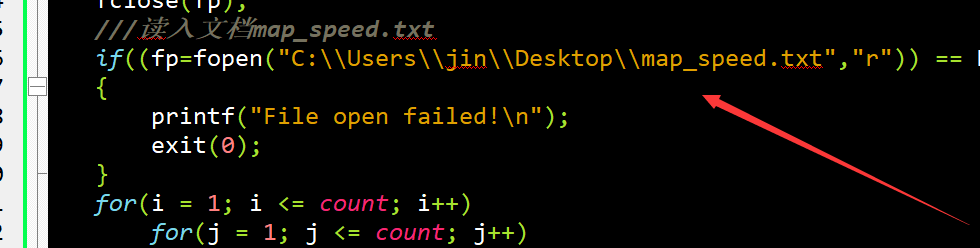运行结果图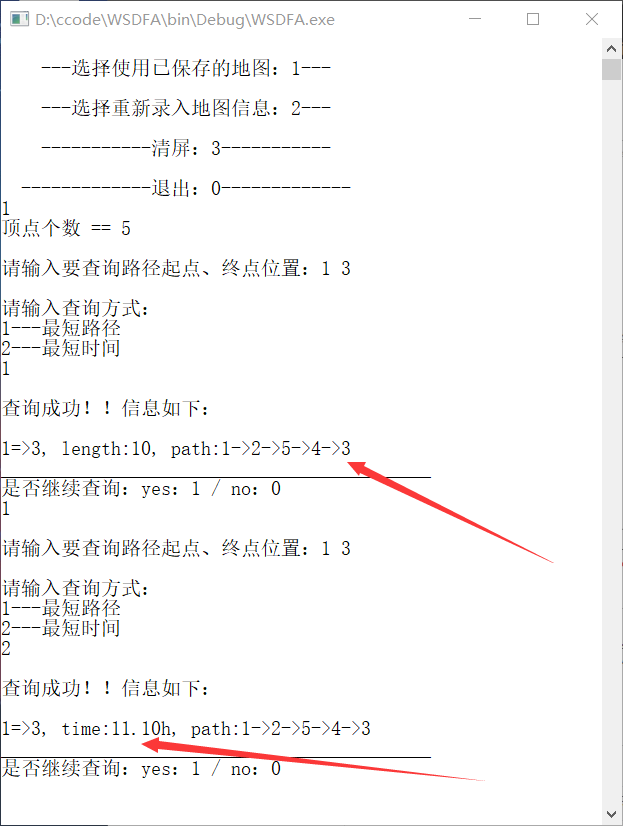我们可以根据之前的测试图，可以看到它的路线

功能2：

录入路线

把代码中的写入的路径更改一下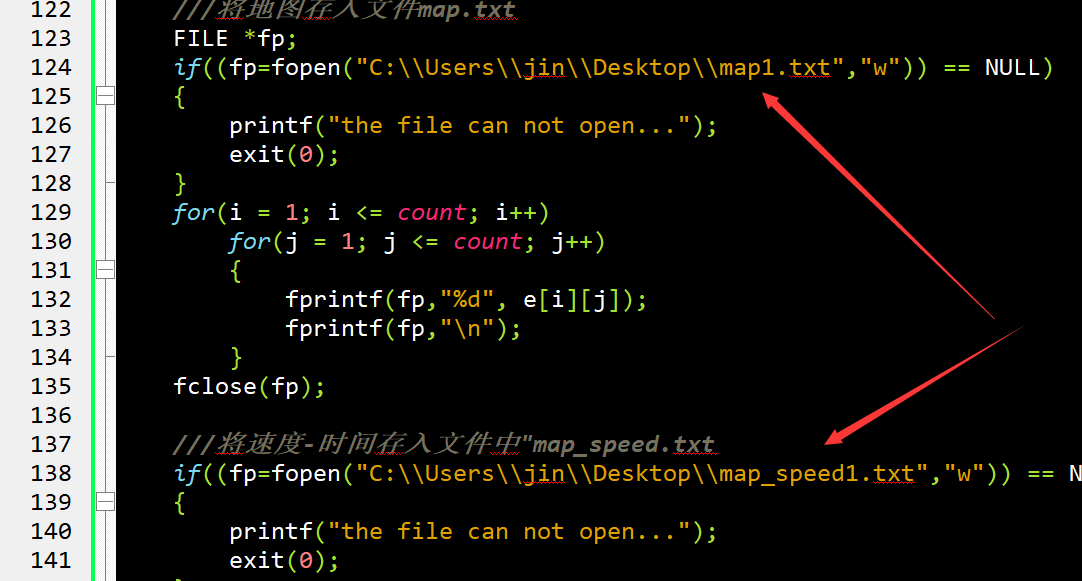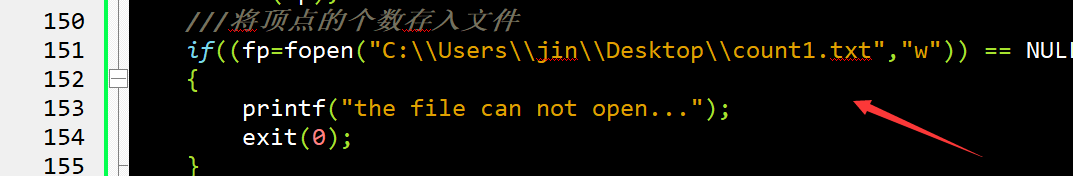这个输入的是两个点是双向都可以通的，点1到点2 的距离是1速度是1，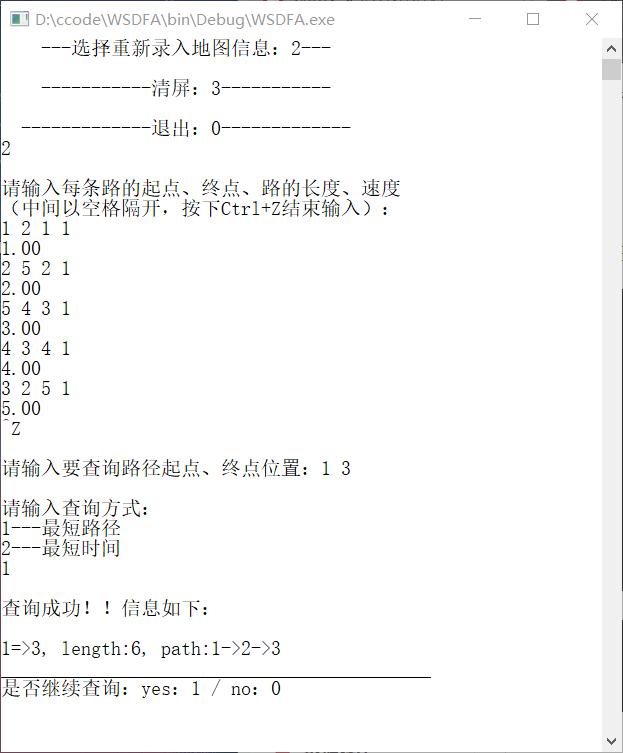思路：很简单，就是用弗罗伊德算法，计算出权值最小的路径，顺便用记录中间的一些中转点,在最后的输出路径中输出出来。

弗罗里达算法思想，想具体知道弗罗里达算法算法自己在网上搜一下吧看吧。

代码

稍微加了一些注释，这个给的代码很简单，应该不用多说吧，不会真的有人看不懂吧

#include <stdio.h>
#include <stdlib.h>
#define inf 99999999
#define max_element 50
int e[max_element][max_element];  //保存地图的数组
int path_e[max_element][max_element], path_t[max_element][max_element];//转折点的路径
double t[max_element][max_element];  //保存地图的速度

void Menu();
void Old_Map();
void New_Map();
void Floyd(int (*e)[max_element],double (*t)[max_element], int n);       //计算最短路径
void Floyd_dist(int (*e)[max_element], int n, int start, int end);
void Floyd_time(double (*e)[max_element], int n, int start, int end);
int main()
{
int Mu=5;
Menu();
while(scanf("%d", &Mu), Mu!=0)
{
switch(Mu)//菜单选项
{
case 1: Old_Map();break;
case 2: New_Map();break;
case 3: system("cls");break;
default:printf("\n请输入正确指令！！！");break;
}

Menu();
}
printf("\n成功退出！");
return 0;
}

void Menu()
{
printf("\n    ---选择使用已保存的地图：1---");
printf("\n\n    ---选择重新录入地图信息：2---\n");
printf("\n    -----------清屏：3-----------\n");
printf("\n  -------------退出：0-------------\n");
}
//使用原有地图
void Old_Map()
{
int i, j;
FILE *fp;
int count = 0;
//读入文档count.txt
if((fp=fopen("C:\\Users\\jin\\Desktop\\count.txt","r")) == NULL)
{
printf("File open failed!\n");
exit(0);
}
fscanf(fp,"%d", &count);//读入点数
fclose(fp);
printf("顶点个数 == %d\n", count);
if(count == 0 )
{
printf("\n信息读入错误！！\n错误原因：没有已保存的地图，请选择重新输入地图信息！！\n");
return ;
}

///读入文档map.txt
if((fp=fopen("C:\\Users\\jin\\Desktop\\map.txt","r")) == NULL)
{
printf("File open failed!\n");
exit(0);
}
for(i = 1; i <= count; i++)
for(j = 1; j <= count; j++)
{
fscanf(fp,"%d", &e[i][j]);
}//读入距离地图，是一个矩阵
fclose(fp);
///读入文档map_speed.txt
if((fp=fopen("C:\\Users\\jin\\Desktop\\map_speed.txt","r")) == NULL)
{
printf("File open failed!\n");
exit(0);
}
for(i = 1; i <= count; i++)
for(j = 1; j <= count; j++)
{
fscanf(fp,"%lf", &t[i][j]);
}//读入时间地图，矩阵
fclose(fp);
///将地图信息打印*************能否采用图形界面将位置信息打印?

Floyd(e,t, count);
}
//新录入地图
void New_Map()
{
//map数组初始化
int i, j;
for(i = 0; i < max_element; i++ )
for(j = 0; j < max_element; j++)
if(i == j)
{
e[i][j] = 0;
t[i][j] = 0;
}

else {
e[i][j] = inf;
t[i][j] = inf;
}

printf("\n请输入每条路的起点、终点、路的长度、速度\n（中间以空格隔开，按下Ctrl+Z结束输入）：\n");
int s, ee, l, speed;
int count = 0;
//地图写入
while(scanf("%d %d %d %d", &s, &ee, &l,&speed) != EOF)
{//开始地，目标地，距离，速度
e[s][ee] = l;
e[ee][s] = l;
t[s][ee] = (l*1.0)/speed;
t[ee][s] = (l*1.0)/speed;
printf("%.2f ", t[s][ee]);
count++;
}
///将地图存入文件map.txt
FILE *fp;
if((fp=fopen("C:\\Users\\jin\\Desktop\\map1.txt","w")) == NULL)
{
printf("the file can not open...");
exit(0);
}
for(i = 1; i <= count; i++)
for(j = 1; j <= count; j++)
{
fprintf(fp,"%d", e[i][j]);
fprintf(fp,"\n");
}
fclose(fp);

///将速度-时间存入文件中"map_speed.txt
if((fp=fopen("C:\\Users\\jin\\Desktop\\map_speed1.txt","w")) == NULL)
{
printf("the file can not open...");
exit(0);
}
for(i = 1; i <= count; i++)
for(j = 1; j <= count; j++)
{
fprintf(fp,"%lf", t[i][j]);
fprintf(fp,"\n");
}//像文件里面写入速度（浮点数）
fclose(fp);
///将顶点的个数存入文件
if((fp=fopen("C:\\Users\\jin\\Desktop\\count1.txt","w")) == NULL)
{
printf("the file can not open...");
exit(0);
}
fprintf(fp,"%d", count);
fclose(fp);
Floyd(e,t, count);
}
//佛洛依德算法
void Floyd(int (*e)[max_element],double (*t)[max_element],int n)
{
int start, end; //起始位置，终点
//初始化记录路径详细信息数组path
int i, j;
//初始化终点***
for( i = 0; i <= n; i++)
for(j = 0; j <= n; j++)
{
path_e[i][j] = j;
path_t[i][j] = j;
}

while(1)
{
printf("\n请输入要查询路径起点、终点位置：");
scanf("%d %d", &start, &end);
if(start > n || end > n)
{
printf("\n出现错误！！！\n错误原因：输入了不存在的顶点！！\n请重新输入！！\n");
printf("\n顶点个数为：%d", n);
continue;
}
int Floyd_xuanze = 0;
printf("\n请输入查询方式：\n");
printf("1---最短路径\n");
printf("2---最短时间\n");
scanf("%d", &Floyd_xuanze);

/**
*异常处理
*/

switch(Floyd_xuanze)
{
case 1: Floyd_dist(e, n, start, end);break;
case 2: Floyd_time(t,n, start, end);break;
default : printf("请输入正确指令！！！\n");break;
}
int temp = 0;
printf("是否继续查询：yes：1 / no：0\n");
scanf("%d", &temp);
if(!temp)
break;
}

}
void Floyd_dist(int (*e)[max_element], int n, int start, int end)
{
int k, i, j;
///佛洛依德算法---距离
for(k = 1; k<=n; k++)//中转点
for(i = 1; i <= n; i++)
for(j = 1; j <= n; j++)
if(e[i][j]>e[i][k]+e[k][j])
{
e[i][j]=e[i][k]+e[k][j];
path_e[i][j] = path_e[i][k];//记录中转点
}

///打印出最短路径及相应路径信息--距离
printf("\n查询成功！！信息如下：\n\n");
printf("%d=>%d, length:%d, ",start, end, e[start][end]);
int v = path_e[start][end];//最开始的中转点
printf("path:%d", start);
while(v!=end)
{
printf("->%d",v);
v = path_e[v][end];
}
printf("->%d", end);
printf("\n___________________________________________\n");
}
void Floyd_time(double (*t)[max_element], int n, int start, int end)
{
int k, i, j;

///佛洛依德算法---时间
for(k = 1; k<=n; k++)
for(i = 1; i <= n; i++)
for(j = 1; j <= n; j++)
if(t[i][j]>t[i][k]+t[k][j])
{
t[i][j]=t[i][k]+t[k][j];
path_t[i][j] = path_t[i][k];
}
///打印出最短路径及相应路径信息--距离
printf("\n查询成功！！信息如下：\n\n");
printf("%d=>%d, time:%.2fh, ",start, end, t[start][end]);
int v = path_t[start][end];
printf("path:%d", start);
while(v!=end)
{
printf("->%d",v);
v = path_t[v][end];
}
printf("->%d", end);
printf("\n___________________________________________\n");
}


代码2（自己写的）

前言

如果你这个看的不是很懂，建议看第一个，

其实也没啥，就是实现一个中文的地点，用map容器进行映射。

其中的难点就是搞了我好久的一个小东西，读取文件，一个词，一个词的读取，搞得我很头疼，不过最后巧妙的解决啦

这个东西如果只用getline(ifs, read, ' ')，读取文件的时候，当读取到第一行最后一个词的时候，会和第二行第一个词一起读，于是乎，可能应该搞了有点长时间把，想了一个巧妙的办法给解决啦，唉这就是生活啊，兵来将挡，水来土掩。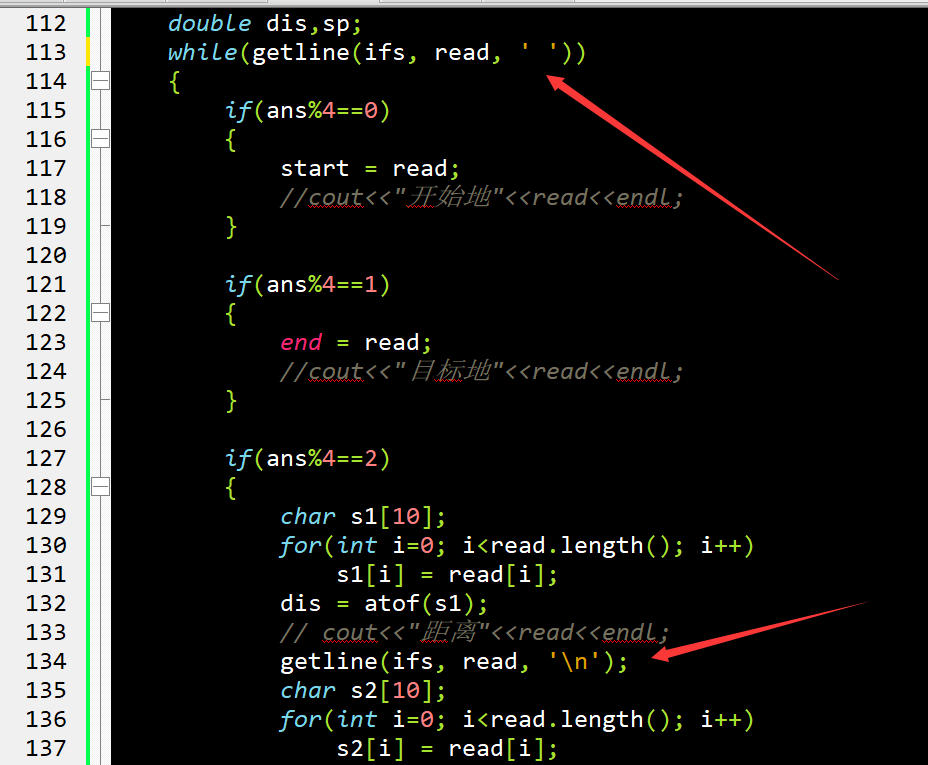整体思路及运行情况

思路：读取文件里面给的路线图，把这些点用弗罗伊德算法，算出最优解，根据所求，输出所要。

主要的亮点就是中文的地点，用了map容器进行一些替换

运行情况：

首先把你的文件调整成ANSI编码，在文件的输入格式是，开始地名，目的地名，距离（浮点型），速度(浮点型)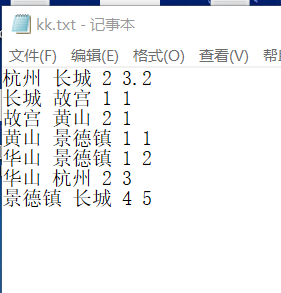这是我的地图，画了一下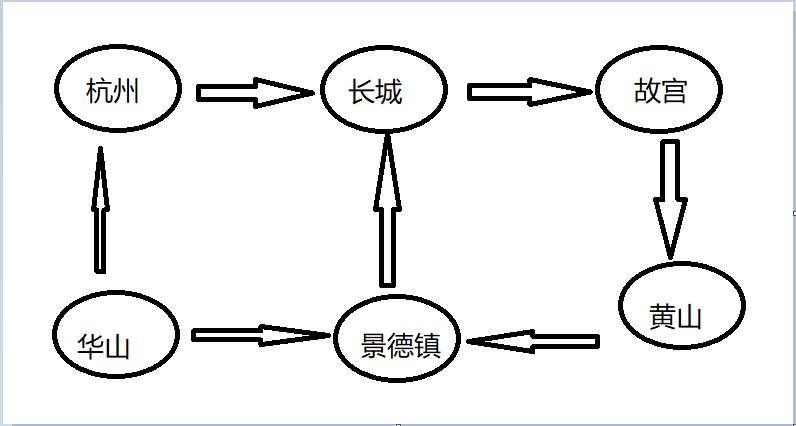开始运行，首先你要知道你的文件的路径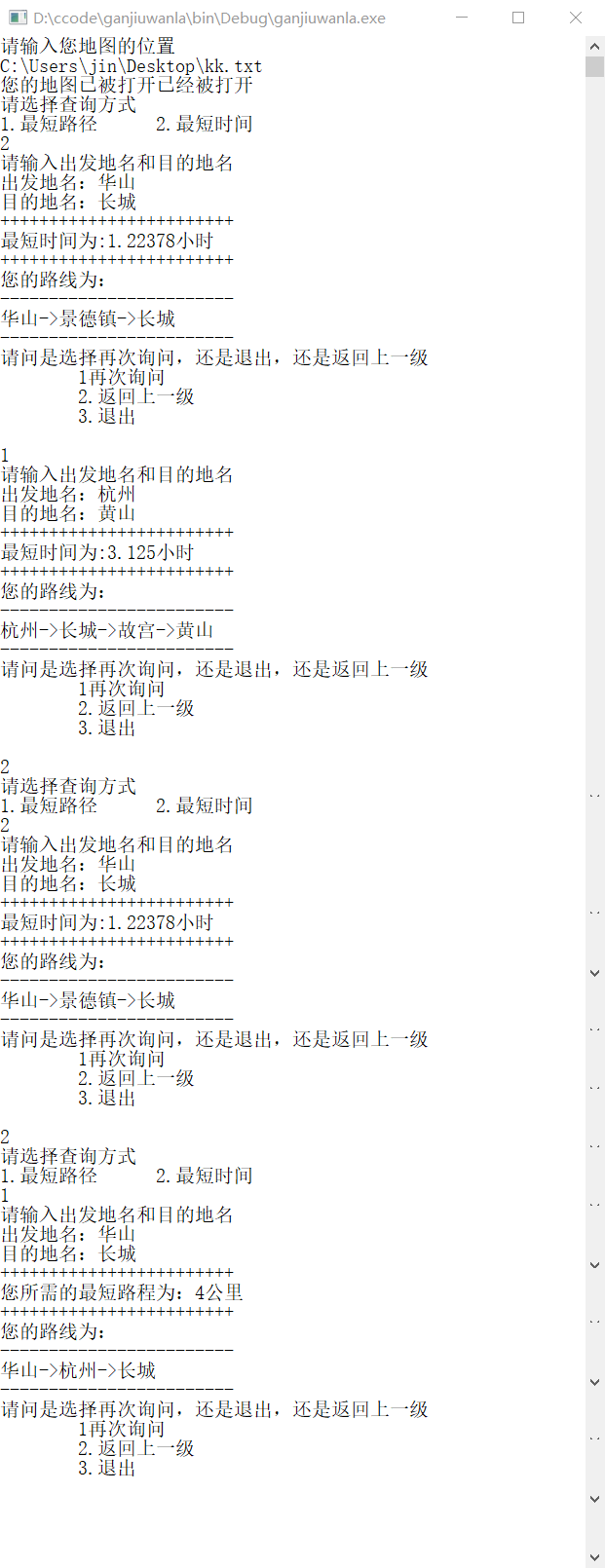代码

#include <bits/stdc++.h>
using namespace std;
typedef long long ll;
map<string,int> pp;
map<int,string> qq;
#define inf 99999999
double dist,timee;
int locations = 0;
void Floyd(double mn,int choose);
void Choose();
void Floyd(double mn,int choose)
{
int path_min;//记录中间转折点
for(int i=1; i<=locations; i++)
for(int j=1; j<=locations; j++)
if(i!=j&&mn[i][j]==0)
{
mn[i][j] = inf;//初始化地图
//cout<<dist[i][j]<<endl;
}

for(int i=1; i<=locations; i++)
for(int j=1; j<=locations; j++)
{
path_min[i][j] = j;//初始化转折点
}
//弗罗里达算法
for(int k = 1; k<=locations; k++)
for(int i=1; i<=locations; i++)
for(int j=1; j<=locations; j++)
{
if(mn[i][j]>mn[i][k]+mn[k][j])
{
mn[i][j] = mn[i][k]+mn[k][j];
path_min[i][j] = path_min[i][k];
}
}
while(1)
{
printf("请输入出发地名和目的地名\n");
printf("出发地名：");
string ss;
cin>>ss;
int st = pp[ss];

printf("目的地名：");
string sss;
cin>>sss;
int ed = pp[sss];
cout<<"++++++++++++++++++++++++"<<endl;
cout<<(choose == 1 ? "您所需的最短路程为：":"最短时间为:");
cout<<mn[st][ed];
cout<<(choose == 1 ? "公里" : "小时")<<endl;
cout<<"++++++++++++++++++++++++"<<endl;
cout<<"您的路线为："<<endl;
cout<<"------------------------"<<endl;
cout<<ss<<"->";
int v = path_min[st][ed];
while(v!=ed)
{
cout<<qq[v]<<"->";
v = path_min[v][ed];
}
cout<<sss<<endl;
cout<<"------------------------"<<endl;
cout<<"请问是选择再次询问，还是退出，还是返回上一级"<<endl;
cout<<"\t1再次询问\n"<<"\t2.返回上一级\n"<<"\t3.退出\n"<<endl;
int c;
cin>>c;
if(c==1) {}
else if(c==2)
Choose();
else if(c==3)
return;
}
}
void Choose()
{
printf("请选择查询方式\n");
printf("1.最短路径\t");
printf("2.最短时间\t\n");
int choose;
cin>>choose;
if(choose==1)
{
Floyd(dist,choose);//最短路程
}
else if(choose==2)
{
Floyd(timee,choose);//最少时间
}
else
{
cout<<"您输入的指令有误，请重新输入"<<endl;
Choose();
}
}
int main()
{
int id=1;
cout<<"请输入您地图的位置"<<endl;
string Path;
cin>>Path;//输入位置
char path;
for(int i=0; i<Path.length(); i++)
path[i] = Path[i];
ifstream ifs(path);
if(ifs!=NULL) cout<<"您的地图已被打开已经被打开"<<endl;
string read;
int ans = 0;
string start,end;//出发地，目标地
double dis,sp;//距离，速度
while(getline(ifs, read, ' '))
{
if(ans%4==0)//读取的第一个词
{
start = read;
//cout<<"开始地"<<read<<endl;
}

if(ans%4==1)//读取的第二个词
{
end = read;
//cout<<"目标地"<<read<<endl;
}

if(ans%4==2)//读取的第三个词和第四个词
{
char s1;
memset(s1,0,sizeof(s1));
for(int i=0; i<read.length(); i++)
s1[i] = read[i];
dis = atof(s1);
//cout<<dis<<"距离"<<read<<endl;
getline(ifs, read, '\n');
char s2;
for(int i=0; i<read.length(); i++)
s2[i] = read[i];
sp = atof(s2);
// cout<<"速度"<<read<<endl;
ans++;
}

//cin>>start>>end>>dis>>sp;
if(ans%4==3)//四个读取完开始储存
{
if(pp[start]==0)//如果这个地点没有被储存，便开始储存
{
pp[start] = id;//地点对应的序号
qq[id] = start;
id++;
}
if(pp[end]==0)
{
pp[end] = id;
qq[id] = end;
id++;
}
dist[pp[start]][pp[end]] = dis;//这段路的而距离
timee[pp[start]][pp[end]] =dis/sp;//这段路的时间
//cout<<dist[pp[start]][pp[end]]<<"--"<<timee[pp[start]][pp[end]]<<endl;
}
ans++;
}

map<string,int>::iterator it;
for(it = pp.begin(); it!=pp.end(); it++)
{
locations++;//统计共有几个地点
//cout<<it->first<<"--"<<it->second<<endl;
}
/*for(int i=1; i<=5; i++)
{
cout<<qq[i]<<endl;
}*/
//cout<<locations<<endl;
Choose();
return 0;
}



展开全文• 煤矿床地表与地质层面模型通常采用TIN表示，交线作为层面模型交叉部分特征描述，在模型构建及后续的应用分析中都具有十分重要作用。根据基于层面模型编制露天煤矿采剥计划需要，提出并实现了一种基于空间索引...TIN 空间索引 碰撞检测 AABB包围盒
• 2.决策树的构建 2.1信息熵 2.2节点构建 2.3决策树的优缺点 3.决策树的编程实现。 3.1 Python编码实现 3.2输出 1.决策树 决策树一般都是自上而下的来生成的。每个决策事件都可能引出两个或多个事件，导致不同...
本文主要介绍决策树的过程：

目录

1.决策树

2.决策树的构建

2.1信息熵

2.2节点构建

2.3决策树的优缺点

3.决策树的编程实现。

3.1 Python编码实现

3.2输出

1.决策树

决策树一般都是自上而下的来生成的。每个决策事件都可能引出两个或多个事件，导致不同的结果，把这种决策分支画成图形很像一棵树的枝干，故称决策树。

基本思想是以信息熵为度量构造一棵熵值下降最快的树，到叶子节点处熵值为0（叶节点中的实例都属于一类）。

2.决策树的构建

2.1信息熵

在决策树构建的过程中，我们需要一个衡量标准来确定每次数据划分所带来的收益，这个标准就是信息熵，以0-1二分类问题为例，衡量一个节点的信息熵公式如下：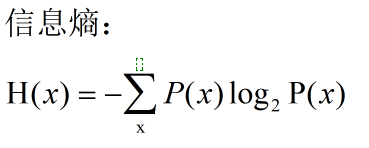信息熵越大代表信息越抽象，决策树中节点信息熵规则为：Entropy（parent）> Entropy（son）> Entropy（grandson）> ...... > 0

2.2节点构建

杜撰了一批数据集，用于具体构建决策树：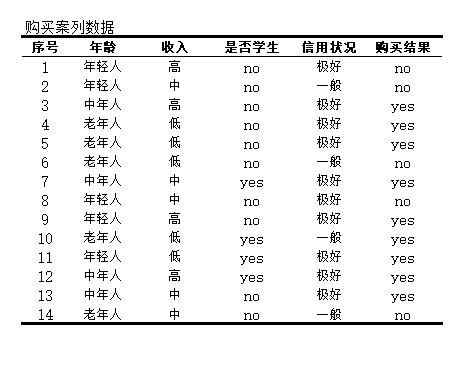决策树中常用ID3归纳算法构建节点，信息获取量过程：Gain(A) = Info(D) - Info_A(D)

通过购买结果来作为分类，看获取了多少信息量。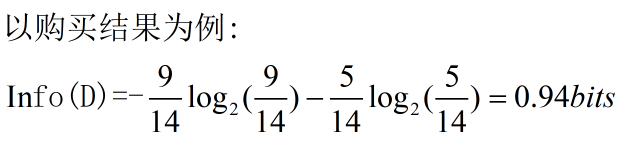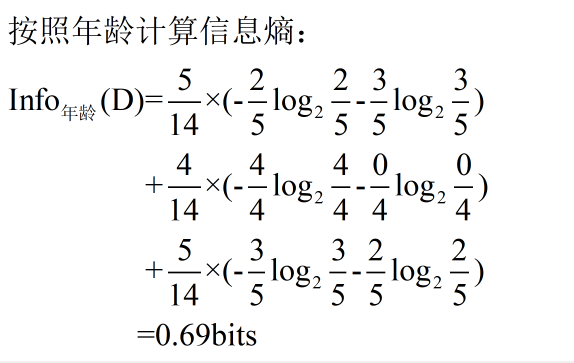故：Gain(年龄) = Info(D) - Info_年龄(D) = 0.94 - 0.69 = 0.25bits

类似地，可以计算出Gain(收入) = 0.03 ，Gain(是否学生) = 0.15，Gain(信用) = 0.05

ID3算法归纳认为，选择年龄作为归纳树的父节点。

父节点的分裂结果为：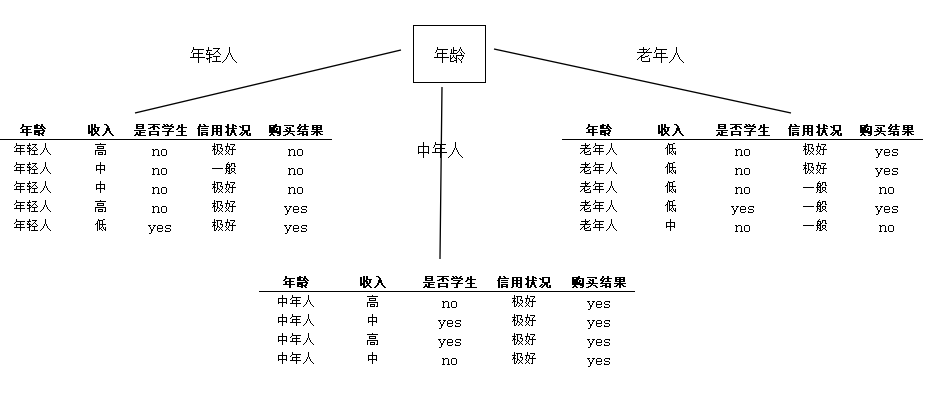对于购买结果为no的情况可以重复上述运算过程，完成一棵完整决策树的构建。

2.3决策树的优缺点

决策树的优点：直观，便于理解，小规模数据集有效；

决策树的缺点：处理连续变量不好，类别较多时，错误增加的比较快。

3.决策树的编程实现。

3.1 Python编码实现

用sklearn实现决策树，代码如下：

from sklearn.feature_extraction import DictVectorizer
import csv
from sklearn import tree
from sklearn import preprocessing
from sklearn.externals.six import StringIO

# Read in the csv file and put features into list of dict and list of class label
allElectronicsData = open(r'train_set.path', 'rt')
reader = csv.reader(allElectronicsData)
headers = next(reader)

print(headers)

featureList = []
labelList = []

for row in reader:
labelList.append(row[len(row)-1])
rowDict = {}
for i in range(1, len(row)-1):
rowDict[headers[i]] = row[i]
featureList.append(rowDict)

print(featureList)

# Vetorize features
vec = DictVectorizer()
dummyX = vec.fit_transform(featureList) .toarray()

print("dummyX: " + str(dummyX))
print(vec.get_feature_names())

print("labelList: " + str(labelList))

# vectorize class labels
lb = preprocessing.LabelBinarizer()
dummyY = lb.fit_transform(labelList)
print("dummyY: " + str(dummyY))

# Using decision tree for classification
# clf = tree.DecisionTreeClassifier()
clf = tree.DecisionTreeClassifier(criterion='entropy')
clf = clf.fit(dummyX, dummyY)
print("clf: " + str(clf))

# Visualize model
with open("Dtree.dot", 'w') as f:
f = tree.export_graphviz(clf, feature_names=vec.get_feature_names(), out_file=f)

oneRowX = dummyX[0, :]
print("oneRowX: " + str(oneRowX))

newRowX = oneRowX
newRowX = 1
newRowX = 0
print("newRowX: " + str(newRowX))

predictedY = clf.predict(newRowX)
print("predictedY: " + str(predictedY))


3.2输出

结果输出为：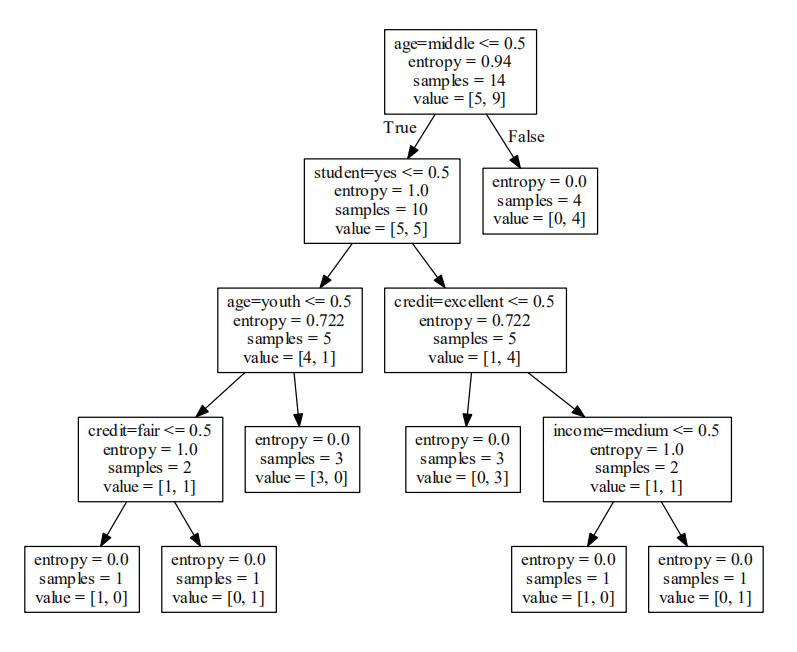展开全文机器学习 决策树 sklearn
• 1．目的：掌握图的存储、构建、搜索等操作和应用，能用最短路径及其搜索等算法编制较综合性的程序，求解最优路线问题，进行程序设计、数据结构和算法设计等方面的综合训练。 2．任务：设计一个城市交通咨询模拟系统...
实验三 图及其应用
实验学时：2                实验类型：综合型
一、目的与任务
1．目的：掌握图的存储、构建、搜索等操作和应用，能用最短路径及其搜索等算法编制较综合性的程序，求解最优路线问题，进行程序设计、数据结构和算法设计等方面的综合训练。
2．任务：设计一个城市交通咨询模拟系统，利用该系统实现至少两种最优决策：最短路程到达、最省时到达等线路规划。
二、内容、要求与安排方式
1．实验内容：
用户驾车出行由于出行目的的不同对道路路线选择的要求也有不同。例如，有的希望在途中的路程尽可能短，有的则可能希望路程中时间最短。为了能满足广大旅客的需求，编制一个城市交通咨询模拟系统，选取城市部分位置、道路抽象为程序所需要图的顶点和边，并以城市道路长度（路程），道路的某时段的速度等信息作为图结点中的弧信息，为旅客提供这两种最优决策的交通咨询。
2．输入和输出：
（1）输入形式：
	构建图时，输入顶点、弧涉及的信息，包括：起始地、目的地、长度、该弧此时间段的平均速度等信息；
	用户或者客户要输入出发地和目的地，并选择何种最优决策的路线规划。
（2）输出形式：根据用户需求输出对应信息
	输出最短路程所需要的路线信息和最短路程；
	输出最短时间所需要的路线信息和最短时间。
3．实验要求：
	实现一个简单的交互式界面，包括系统菜单、清晰的输入提示等。
	根据输入的交通图数据，以图形化形式把交通图显示在屏幕上。
	以图形化形式把最优路线显示在屏幕上。
	能够上机编辑、调试出完整的程序。
4．实验安排方式：
	在实验课前编写出完整程序，在实验课时进行调试；
	每组1人，独立完成上机实验。
三、注意事项：
	请在实验报告中详细描述最优决策算法思想。
参考：交通路线图示例数据
假定根据城市道路抽象为如下图，起始地为V1顶点，目的地为V9顶点，对用弧上的距离和此时间段平均速度等信息如下表所示：
弧	a1	a2	a3	a4	a5	a6	a7	a8	a9	a10	a11
距离
（Km）	12	8	6	5	8	7	5	6	10	6	8
速度
（Km / h）	40	50	60	20	25	55	20	20	40	30	25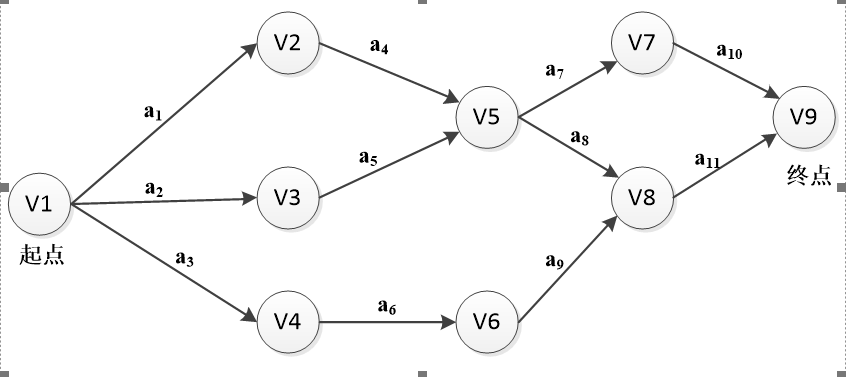【实现思路】
分别存储两种权值，分别对两种权值进行dijkstra
避免了使用高级数据结构，直接二维数组打一个算法模板即可。
【实现方法】
dijkstra
//solve.h
#ifndef SOLVE_H_INCLUDED
#define SOLVE_H_INCLUDED

typedef struct{
int start, eend;
int len, speed;
}Node;

#endif // SOLVE_H_INCLUDED


//mian.cpp
/**
author:mmciel
time:2019年5月16日22:35:55
问题描述：
多权值、单终点
Dijkstra 算法
*/
#include <bits/stdc++.h>
using namespace std;
const int inf = 0x3f3f3f3f;
const int maxn =105;
int lmap[maxn][maxn];//路径作为权值的地图
double tmap[maxn][maxn];//时间作为权值的地图
int n,m;//节点数与路径数

int disl[maxn];//路径作为权值的最短路径表
int prevl[maxn];//路径作为权值的最短路径还原表
bool usel[maxn];//路径作为权值的是否访问标记

double dist[maxn];//时间作为权值的最短路径表
int prevt[maxn];//时间作为权值的最短路径还原表
bool uset[maxn];//时间作为权值的是否访问标记

int start,eend;//用户输入的起始地点与终止节点
vector<int> path;//路径还原的临时存储

vector<int> getPath(int t,int o);//路径的临时记录
void welcome();//欢迎界面
void InputData();//输入数据
void setData();//使用内部生成的数据
void dijkstral(int s);//对路径进行dijkstra算法
void dijkstrat(int s);//对时间（double值）进行dijkstra算法
void init();//清空地图、访问标记、路径还原、临时存储等变量的值
int main()
{
int order = -1;
bool flag = true;
while(1){
welcome();
cout<<"请输入命令：";
cin>>order;
switch(order){
case 1:
init();
setData();
break;
case 2:
init();
InputData();
break;
case 3:
cout<<"请输入起点、终点：";
cin>>start>>eend;
dijkstral(start);
cout<<"最短路径：";
cout<<disl[eend]<<endl;
path.clear();
path = getPath(eend,1);
for(int i=0; i<path.size(); ++i)
cout<<path[i]<<"->";
cout<<endl;
break;
case 4:
cout<<"请输入起点、终点：";
cin>>start>>eend;
dijkstrat(start);
cout<<"最短时间：";
cout<<dist[eend]<<endl;
path.clear();
path = getPath(eend,2);
for(int i=0; i<path.size(); ++i)
cout<<path[i]<<"->";
cout<<endl;
break;

break;
default:
cout<<"退出..."<<endl;
flag = false;
break;
}
if(!flag){
break;
}
}
return 0;
}

void setData(){
n = 9;
m = 11;
lmap = 12;
lmap = 8;
lmap = 6;
lmap = 5;
lmap = 8;
lmap = 7;
lmap = 5;
lmap = 6;
lmap = 10;
lmap = 6;

tmap = 12.0/40;
tmap = 8.0/50;
tmap = 6.0/60;
tmap = 5.0/20;
tmap = 8.0/25;
tmap = 7.0/55;
tmap = 5.0/20;
tmap = 6.0/20;
tmap = 10.0/40;
tmap = 6.0/30;
cout<<"写入成功"<<endl;
}



展开全文• 在分析Web应用程序语句特征基础上，定义了由页面引起Web页面间各种依赖关联，并构建了Web应用结构依赖WAStrDG。基于WAStrDG所实现的Web结构切片算法有助于获取Web结构层次信息，可以有效提高Web测试和...
• Shape Visualizer旨在作为一种探索性/教育... 主网站被构建为Wiki，以收集有关各种已实现算法的信息和示例。 该应用程序及其Wiki组合旨在构成一个用于多尺度分析不错教学方法小工具包。 随意贡献代码或Wiki内容！开源软件
• 为获得复杂混沌信号源,构建...仿真结果表明,实现电路相轨迹与系统相轨迹完全一致,证实所构建的电路系统为超混沌系统,且该系统可以作为混沌加密通信信号源实现对信号进行加密,为信息加密提供了一种新方法。
• （３）实现了环境稠密地图的构建。本文利用视觉ＳＬＡＭ系统前端获得的关键帧与相机位姿信息，构建环境的稠密点云地图和八叉树地图。实验表明，稠密点云地图具有较好的可视化效果；八叉树地图能够提供空间是否被物体...
• 针对分类器的构建，在保证基分类器准确率和差异度的基础上，提出了采用差异性度量特征选择的多分类器融合算法(multi-classifier fusion algorithm based on diversity measure for feature selection, MFA-DMFS)。...特征选择 图像检索 PCA
• （1）熟练掌握图的邻接矩阵与邻接表存储结构及其应用； （2）能设计出基于两种存储方法的应用程序； （3）理解并掌握最短路径算法的基本思想及其算法方法的实际应用。 实验内容： 构建一个校
数据结构C语言版 - 图的应用
前言：

大二本科计算机科学与技术程序员一枚，总结几篇课后实验内容，希望可以帮助到大家。
软件：Devc++

实验目的：
通过实验掌握图的基本存储原理，能够利用图模型存储数据并实现对图的两点间的最短路径的求取过程，巩固所学的基本概念和方法，掌握必要的图模型数据结构的程序设计技术。
（1）熟练掌握图的邻接矩阵与邻接表存储结构及其应用；
（2）能设计出基于两种存储方法的应用程序；
（3）理解并掌握最短路径算法的基本思想及其算法方法的实际应用。

实验内容：

构建一个校园交通查询系统程序。能够规划出任意出发地和目的地之间的最短路径。

实验步骤:
（1）将校园中的重要地点（如教学楼、宿舍楼、餐厅、图书馆等主要地点位置以及主要的道路和路口等联系起来并绘制草图，然后将其抽象为图中的结点（序号）、边，道路中各相邻结点间的近似距离作为边上的权值，形成图结构）；
（2）对上述图结构采用邻接矩阵方法进行存储，图中结点的实际名称和图序号之间的对应关系可以另外通过建立一个一维数组对应存储（名称和序号能够实现相互转换）；
（3）交通查询：输入一个图中起点位置名称（可由程序转换为结点序号）以及要到达的目的地位置名称（可转换为结点序号），就可通过Floyd算法规划出该两点间的一条最短路径并输出该路径中间所经过的主要位置（结点）的名称序列以及该路径的距离。

实验难点:
本实验的难点在于两点：
1.图的邻接矩阵的建立，有两张方式，一种是通过键盘输入相关地点和权值建立，另一种是通过读取文本文件的方式建立。我采用的是后者。
2.Floyd算法的输出。

实验过程
先看一下程序要的结果图：建立图的邻接矩阵
先看一下通过读取文本内容的txt文件的存放路径以及内容。这个txt文本文件一定要放到当前源文件的目录下，具体代码后面会讲到。
txt文本内容说明
6 8 : 要建立的图共有6个顶点和8条边
各个序号后面跟着的地点名称为 每个顶点代表的地点名称，因为题目要求实现结点序号和地点名称相互转化，我采用了这种方式，当然，你也可以在代码中自己创建一个一维数组进行保存。
0     1 50 ： 代表0号结点到1号结点的权值为50
ctrl+S保存后我们的txt文件就可以放到那里不用动了
                                     ==接下来上代码==

需要用到的头文件
#include <stdio.h>
#include <stdlib.h>
#include <string.h>
#include <conio.h>
#include <Windows.h>

常量定义
#define M 100   //图最大的结点数目
#define INFINITY 100000  //无穷

typedef int EdgeType;
typedef int Distance[M][M];   /* 距离向量类型*/
typedef int Path[M][M];   /* 路径类型*/

用到的结构体
typedef struct {
char name;               //学校地名的名称
int id;                      //地名的编号
}VextexType;

typedef struct{
VextexType vertex[M];    //结点信息
EdgeType arc[M][M]; //图的边的信息
int n, e;    //图的结点数和边数
}MGraph;

函数声明
void  Menu(); //主界面函数
void Create(MGraph *G); //用读取文件的方式建立图的邻接矩阵
int NameId(MGraph G, char *loc); //通过学校地点名称查找景点的编号
void Floyd(MGraph G, char *loc1, char *loc2, Path path, Distance distance); //Floyd算法
void PrintPath(int u, int v, int path[][M], MGraph G, Distance d); //输出最短路径所经过的结点以及最短距离
void printnum(MGraph *G); //输出编号以及地点名称
void printSchool(MGraph *G); //输出学校地点的图邻接矩阵
void Exit(); //退出程序

完整代码
#include <stdio.h>
#include <stdlib.h>
#include <string.h>
#include <conio.h>
#include <Windows.h>

#define M 100   //图最大的结点数目
#define INFINITY 100000  //无穷

typedef int EdgeType;
typedef int Distance[M][M];   /* 距离向量类型*/
typedef int Path[M][M];   /* 路径类型*/

typedef struct {
char name;               //学校地名的名称
int id;                      //地名的编号
}VextexType;

typedef struct{
VextexType vertex[M];    //结点信息
EdgeType arc[M][M]; //图的边的信息
int n, e;    //图的结点数和边数
}MGraph;
/*主界面函数*/
void Menu(){
printf("\n\n\t\t\t 欢迎使用中北大学信息商务学院校园导游系统");
printf("\n\t\t\t*******************************************\n\n\n");
printf("\t\t\t\t请选择您需要的功能\n\n");
printf("\t\t\t\t1.查询两个点之间的最短路径(Floyd)\n\n");
printf("\t\t\t\t2.输出编号以及地点名称\n\n");
printf("\t\t\t\t3.输出学校地图的邻接矩阵(无向图)\n\n");
printf("\t\t\t\t4.退出程序\n");
printf("\n\n\t\t\t*******************************************\n\n");
}
/*用读取文件的方式来建立图的邻接矩阵*/
void Create(MGraph *G){
int i, j, k, w;

FILE *rf;
rf = fopen("D:\\Devc++Work\\Graph\\g1.txt", "r"); //这里的路径为txt文本的路径
if (!rf){
printf("error!");
return;
}
if (rf)
{
fscanf(rf, "%d%d", &G->n, &G->e);
for (i = 0; i<G->n; i++)
{
fscanf(rf, "%d%s", &G->vertex[i].id, G->vertex[i].name);
}

for (i = 0; i<G->n; i++)
{
for (j = 0; j<G->n; j++)
{
if (i == j) G->arc[i][j] = 0;
else G->arc[i][j] = INFINITY;
}
}
for (k = 0; k<G->e; k++)
{
fscanf(rf, "%d%d%d", &i, &j, &w);
G->arc[i][j] = w;
G->arc[j][i] = w;
}
fclose(rf);
}
else G->e = 0;
}
/*通过学校地点名称查找景点的编号*/
int NameId(MGraph G, char *loc) {
int i;
int flag = -1;
for (i = 0; i < G.n; i++) {
if (strcmp(G.vertex[i].name, loc) == 0) {
flag = i;
break;
}
}

return flag;
}

/*Floyd算法*/
void Floyd(MGraph G, char *loc1, char *loc2, Path path, Distance distance){
int l1, l2;

l1 = NameId(G, loc1);
l2 = NameId(G, loc2);

int i, j, k;
//初始化distance数组和path数组
for (i = 0; i < G.n; i++) {
for (j = 0; j < G.n; j++) {
distance[i][j] = G.arc[i][j];
path[i][j] = -1;

}
}
//三层循环，寻找最短路径
for (k = 0; k < G.n; k++){ //这层代表中间点
for (int i = 0; i < G.n; i++) {
for (int j = 0; j < G.n; j++) {
if (distance[i][j] > distance[i][k] + distance[k][j]) {
distance[i][j] = distance[i][k] + distance[k][j]; //顶点之间的距离
path[i][j] = k;
}
}
}
}
}
/*输出最短路径所经过的结点以及最短距离*/
void PrintPath(int u, int v, int path[][M], MGraph G, Distance d) {
int mid;

if (path[u][v] == -1) {
//直接输出，没有中间点
printf("<%s,%s>", G.vertex[u].name, G.vertex[v].name);
}
else { //有中间点
mid = path[u][v];
PrintPath(u, mid, path,G,d);
PrintPath(mid, v, path,G,d);
}
}
//输出编号以及地点名称
void printnum(MGraph *G)
{
int i = 0;
printf("\n");
for (i = 0; i<G->n; i++)
{
printf("                     地点编号及名称:%d\t%s\n", G->vertex[i].id, G->vertex[i].name);
printf("\n");
}
}

/*输出学校地点的地图邻接矩阵*/
void printSchool(MGraph *G){
int i, j;
printf("                     学校地图的邻接矩阵为:\n");
for (i = 0; i < G->n; i++)
{
for (j = 0; j < G->n; j++)
{
if (G->arc[i][j] == INFINITY)
printf("          ∞\t");//用∞代表俩顶点之间无联系
else
printf("          %d\t", G->arc[i][j]);
}
printf("\n");
}
}

/*退出程序的函数*/
void Exit(){
for (int i = 2; i >= 1; i--) {
printf("                     程序正在退出，倒计时：%d秒\n", i);
Sleep(1000);
}
exit(0);
}
/*主函数入口*/
int main() {
Path p;
Distance d;
MGraph G;
Create(&G);

while (1){
int choice;
char loc1 = { 0 };
char loc2 = { 0 };

Menu();
printf("                     请输入你想要的的操作:");
scanf("%d", &choice);
switch (choice)
{
case 1:

printf("                     请输入您想查找最短路径的两个地点的名称:");
scanf(" %s %s", &loc1, &loc2);
if (NameId(G, loc1) == -1 || NameId(G, loc2) == -1){
printf("学校地点输入错误!\n");
}
else{
Floyd(G, loc1, loc2, p, d);
int l1 = NameId(G, loc1);
int l2 = NameId(G, loc2);
printf("                     %d号-%d号 最短距离为:%d米 \n", l1, l2, d[l1][l2]);
printf("                     最短路径为:");
PrintPath(l1,l2,p,G,d);
}
break;
case 2:
printnum(&G);
break;
case 3:
printSchool(&G);
break;
case 4:
Exit();
break;

default:
break;
}

}
return 0;
}

总结
第一次写博客，有改进的地方希望可以一起交流，热爱学习，好好生活。


展开全文编程语言 数据结构 c语言
• 为获得复杂混沌信号源，构建四维超...仿真结果表明，实现电路相轨迹与系统相轨迹完全一致，证实所构建的电路系统为超混沌系统，且该系统可以作为混沌加密通信信号源实现对信号进行加密，为信息加密提供了
• 提出一种非相干字典学习及稀疏表示方法...采用合成雨与真实雨算法进行验证，实验结果表明，算法所学习非相干字典具有较好稀疏表示性能，去雨后图像雨线残留较少，边缘细节保持较好，视觉效果更为清晰自然。字典学习 稀疏表示
• 通用盲检测优点是对多种类型隐写算法有效，适应性强，经过样本学习，对未知算法或新算法有效，具有泛化能力，期间尝试应用Libjpeg提取JPEG质量因子来提高检测正确率。同样，通用盲检测也有缺点，相对于针对...隐马尔科夫模型
• 文章目录堆及其应用插入调整删除调整应用合并有序小文件高性能定时器求topK大求中位数 堆及其应用 ​ 堆是一个完全二叉树，分为大顶堆和小顶堆。大顶堆每一个节点值都必须大于等于其子树中每个节点值，小顶堆...
• 由于该方法有在聚类上无教师监督独特优点，并且通过对人脑MR 聚类和分割两个实验，证明了该分割算法比以往分割算法在具体应用上都有一定提高。 灰色人工神经网络人口总量预测模型及应用.pdf ...matlab
• 第6章改进广义预测控制算法分析与实现 6.1预测控制 6.1.1基于CARIMA模型JGPC 6.1.2基于CARMA模型JGPC 6.2神经网络预测控制MATLAB实现 第7章SOFM网络算法分析与应用 7.1SOFM网络生物学基础 7.2SOFM...
• 研究现状及算法实现进行了系统分析和深入研究，取得了一系列研究成 果。本文研究工作丰富和完善了数字水印技术，对多媒体信息版权保护发展 起到了积极推动作用，尤其是数字地图水印开拓性研究将进一步...
• seqwish实现了从序列之间的成对比对到编码序列及其比对的变异图的无损转换。 作为输入，我们通常采用所有对所有对齐方式，但是可以以特定于应用程序的方式定义对齐方式集的确切结构。 该算法使用一系列磁盘支持的...C++
• 由于该方法有在聚类上无教师监督独特优点，并且通过对人脑MR 聚类和分割两个实验，证明了该分割算法比以往分割算法在具体应用上都有一定提高。 灰色人工神经网络人口总量预测模型及应用.pdf ...matlab
• 提高篇 第1章 精通“图像特征提取” 1.1 图像多分辨率金字塔 1.1.1 浅析“图像金字塔” 1.1.2 例程一点通 1.1.3 典型“图像金子塔 ...3.1 图像去噪技术及其实现 3.1.1 什么是“图像噪声” 3.1.2 图像去噪常用方法
• 随着虚拟现实及其相关技术发展,数字地球、数字中国、数字城市越来越受到人们关注,虚拟场景建模技术研究成为近年来国内外一个研究热点,具有十分广泛实用价值和应用前景。目前VRML(Virtual Reality Modeling ...
• 以图形和文字等形式形象得描述系统整体物理架构模型，解释系统组成结构，重要节点，及其之间物理联系，具体框架见3-1-1所示 3-1-1 四川疫情可视化，系统功能框架设计 3.2项目技术框架设计 ​ 分析爬虫项目...Python
• OCG编译算法会仔细考虑每一个变量各种情况，加上将pointer value分配给指针情况（无论通过功能回归、功能参数传递直接分配，还是通过其它这阵非直接分配），构建数据引用，也就是指针引用（Pointer ...Cypress
• 针对现有棋盘格角点检测算法存在精度不足问题,提出一种基于Hough变换和圆形模板高精度棋盘格角点检测算法:首先,利用Hough变换和棋盘格直线分布特征获取有效直线,构建棋盘格角点,实现角点粗略定位;其次,构建...